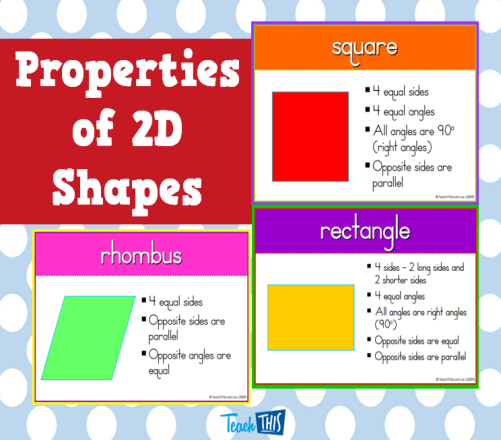# What Do You Know About Properties Of Shape?

10 Questions | Total Attempts: 109SettingsEvery object has certain characteristics and essential to them, for example, their weight, length how they look like among other entity, take a quiz and learn about properties of the shape.

• 1.
A particular shape with 6 equal sides, 6 equals angles of 120 degrees, 6 lines of symmetry, 0 perpendicular lines and 3 pairs of parallel line is called?
• A.

Nanogon

• B.

Polygon

• C.

Hexagon

• D.

Tetrahedron

• 2.
A shape with 0 pairs of parrallel lines, 0 perpendicular lines, 5 lies of symmetry, 5 equal angles of 108 degree and 5 equal sides is called?
• A.

Cone

• B.

Pentagon

• C.

Triangle

• D.

Circle

• 3.
A particular triangle with 0 pairs of parallel lines, 0 perpendicular lines, 3 lines of symmetry, 3 equals angles of 60 degrees and 3 equal sides is called?
• A.

Bilateral

• B.

Equilateral

• C.

Triangle

• D.

Isosceles

• 4.
A regular shape with 7 equal sides, 0 pairs of parallel lines, 0 perpendicular lines, 7 lines of symmetry and 7 equal angles of 128.52 degrees is called?
• A.

Circle

• B.

Cube

• C.

Heptagon

• D.

Tetrahedron

• 5.
3-Dimensional shapes are superb and give us the clear picture of a particular object which is close to real life, it can also be referred as?
• A.

3-Di

• B.

Three D

• C.

3Ds

• D.

3D

• 6.
A particular three-dimensional shape with 6 faces, 8 vertices on all sides and 12 edges around it is called?
• A.

Polygon

• B.

Circle

• C.

Cone

• D.

Cube

• 7.
2 Dimensional shapes give us a theoretical and diagrammatical representation of an object, they are also referred to as?
• A.

2D

• B.

2 Dimension

• C.

2Ds

• D.

(d) None of the above

• 8.
A particular shape with four-sided shapes in which opposite sides are parallel and equal, opposite angle is also equal is referred to as?
• A.

Triangle

• B.

Trapezoid

• C.

Parallel line

• D.

Parallelogram

• 9.
A parallelogram with four equal sides, opposite angles are also equal with zero perpendicular lines is referred to as?
• A.

Rhombus

• B.

Cone

• C.

Rectangle

• D.

Triangle

• 10.
A particular 3-dimensional shape with 6 faces to it, 12 edges around it and 8 vertices on all side is referred to as?
• A.

Drone

• B.

Cuboid

• C.

Sphere

• D.

Cone

Related TopicsBack to top